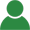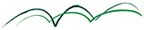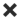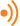ErrorHome Courses Course Calendar Teaching Team About Us Resources Contact

Can't travel? Don't want to travel? LFS Livebrings the class to you!

• Live interactive training from world renowned practitioners in the comfort of your own home
• Real classroom experience without the inconvenience of travel
• World class teaching from the comfort of your preferred location

Course Calendar

# Intermediate Mathematics: Understanding Stochastic Calculus

## Day One

### Probability Theory

• Random variables, independence and conditional independence. Discrete random variables: mass density, expectation and moments calculation
• Conditional discrete distributions, sums of discrete random variables
• Continuous random variables; Probability density function, cumulative probability density function; Expectation and moments calculation; Conditional distributions and conditional expectation; Functions of random variables

Examples: Normal distribution, gamma distribution, exponential distribution, Poisson distribution

Exercise: Properties of the gamma distribution and the log-normal distribution

Workshop: Multivariate normal distributions. Linear transformations. Counter-example

• Generating functions. Moment generating functions. Characteristic functions
• Convergence theorems: the strong law of large numbers, the central limit theorem

Examples: Characteristic functions of Bernoulli, binomial, exponential distributions

Exercise: Moment generating functions and characteristic functions of Poisson, normal and multivariate normal distributions

### Markov Chains

• Discrete time Markov chains, the Chapman-Kolmogorov equation
• Recurrence and transience. Invariance
• Discrete martingales. Martingale representation theorem. Convergence theorems

Examples: Random walks: simple, reflected, absorbed

Workshop: Pricing European options within the Cox-Ross-Rubinstein model

• Continuous time Markov chains. Generators
• Forward/backward equations. Generating functions

Example: The Poisson process

Exercise: Superposition of Poisson Processes. Thinning

## Day Two

### Stochastic Calculus

• The Wiener process. Path properties. Monte Carlo simulation
• Gaussian processes. Diffusion processes

Examples: The Wiener process with drift. The Brownian Bridge

Exercise: The Geometric Brownian Motion. Properties of its distribution (moments)

• Semi-martingales. Stochastic integration
• Ito's formula. Integration by parts formula

Workshop: Multivariate normal distributions. Linear transformations. Counter-example

Examples: Characteristic functions of Bernoulli, binomial, exponential distributions

Exercises: Moment generating functions and characteristic functions of Poisson, normal and multivariate normal distributions

### Stochastic Differential Equations

• Stochastic differential equations. Existence and uniqueness of solutions. Equations with explicit solutions
• The Markov property. Girsanov's theorem

Exercise: The Vasicek model. Connection with the O-U process. Mean. Variance. Covariance. Pricing zero-coupon bonds

Workshop: The Cox Ingersoll Ross Model. Connection with the O-U process. Properties of its distribution (mean variance, covariance). Pricing zero-coupon bonds